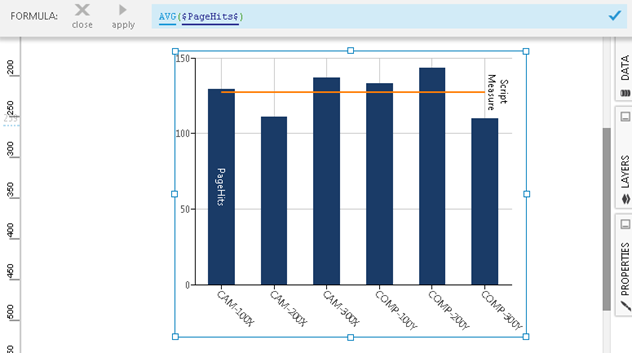# Mean (Simple Average)

Contents[Hide]

The AVG function computes the mean (or simple average) of a set of input values by calculating the total (sum) and deviding it by the number of values.Mean (Simple Average)

## 1. Syntax

```AVG(d0,Alignments...)
```

## 2. Input

The AVG function requires the following input:

• d0 - The set of data values for which the mean is calculated.

## 3. Parameters

The AVG function requires the following parameter:

• Alignments... (One or More, Optional) – Hierarchy placeholders to be used as the alignment axis.

## 4. Output

The AVG function generates the following output:

• A single value representing the mean of the input data values.

## 5. See also

Dundas Data Visualization, Inc.
500-250 Ferrand Drive
Toronto, ON, Canada
M3C 3G8

North America: 1.800.463.1492
International: 1.416.467.5100

Dundas Support Hours: 7am-6pm, ET, Mon-Fri